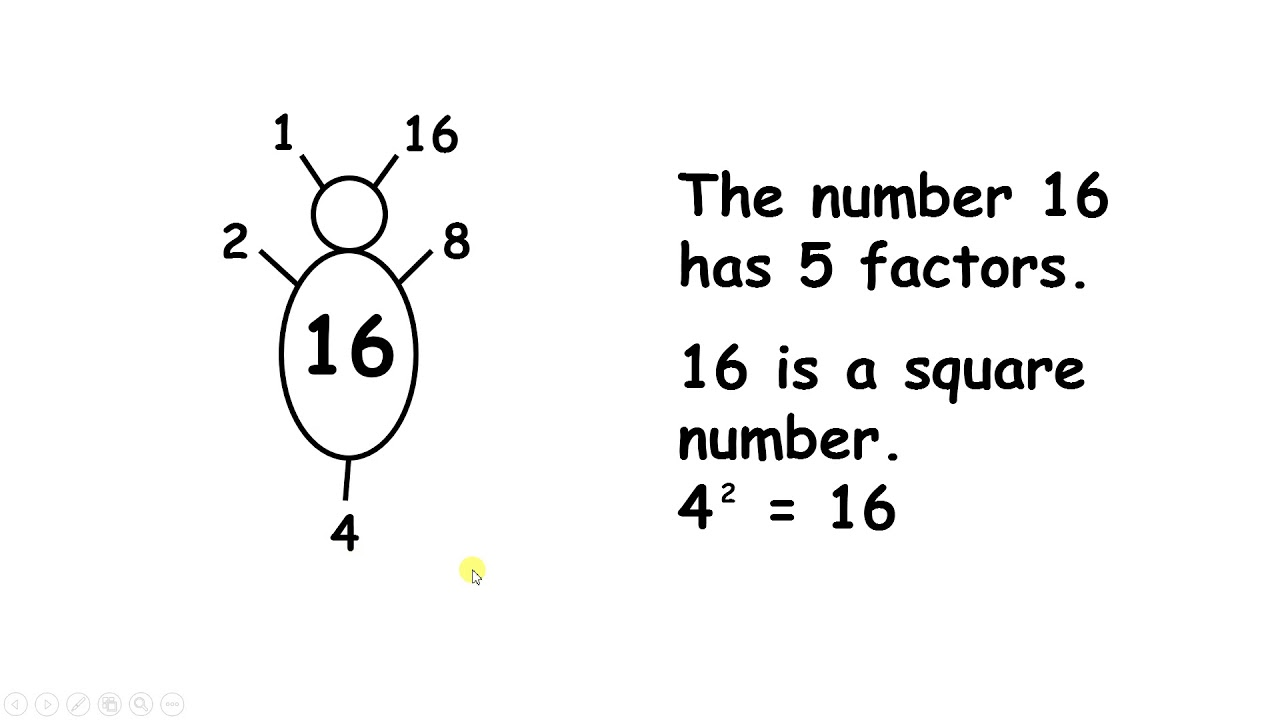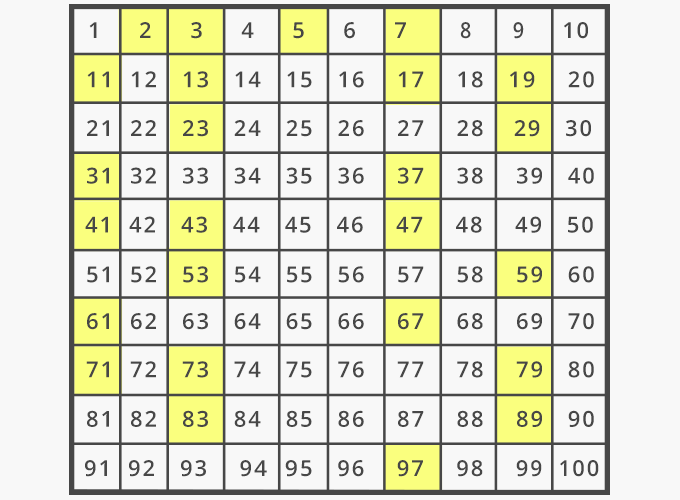# Y6 Maths: Factors and Prime numbers

Factors are whole numbers (integers) which multiply with another number to make an answer (called a multiple).  So for example, the factors of 12 are 1, 2, 3, 4, 6 and 12 because the number 12 can be made by multiplying the following pairs:

1 x 12 = 12

2 x 6 = 12

3 x 4 = 12

We have been using factor bugs to calculate factors of numbers.  These work in a sequence (starting with 1) to help us work out what the factors of a number are.  By working in order (1, 2, 3, 4...) we can make sure that we don't miss any factors.  The numbers at the head are always the numbers 1 and the number itself, as these are the prime factors (see below).  The factor pairs are on opposite sides of the bug.  Once you pass the halfway mark, there are no more factors to find.  If a number is a square number (it has a factor that multiplies by itself: for example 4 x 4 = 16) the factor is only written once, as a tail. Once you get to the tail, there are no more factor pairs to find.Prime numbers are numbers which only have two factors: one and the number itself.  The number one is not a prime number as it only has one factor (1 x 1 = the number 1).